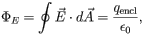# Problem: To understand the meaning of the variables in Gauss's law, and the conditions under which the law is applicable.Gauss's law is usually writtenwhere ε0 = 8.85 x 10-12 C2/(N • m2) is the permittivity of vacuum.How should the integral in Gauss's law be evaluated?a) around the perimeter of a closed loopb) over the surface bounded by a closed loopc) over a closed surface

###### FREE Expert Solution

a)

Around the perimeter of a closed loop, there is no field lines enclosed through the surface.

Gauss law cannot be evaluated around the perimeter of a closed-loop.###### Problem Details

To understand the meaning of the variables in Gauss's law, and the conditions under which the law is applicable.

Gauss's law is usually writtenwhere ε= 8.85 x 10-12 C2/(N • m2) is the permittivity of vacuum.

How should the integral in Gauss's law be evaluated?

a) around the perimeter of a closed loop

b) over the surface bounded by a closed loop

c) over a closed surface